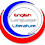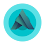# MATHEMATICS: First Term's Scheme of Work for SSS 1 - 3

## MATHEMATICS: First Term's Scheme of Work for SSS 1 - 3

17
Here is the Mathematics scheme of work for Senior Secondary Schools (SSS) 1 – 3.

Mathematics Scheme of Work for SSS 1

Week 1: NUMBER BASES
i. Decimal Base (Base 10) and other bases, e.g., base 2 (binary) etc.
ii. Conversation from Base 10 to other bases.
iii. Conversation from other bases to Base 10.

Week 2: NUMBER BASES II
i. Problem solving, addition, subtraction, multiplication and division of number in the various bases.
ii. Conversion of decimal fraction in one base to base 10.
iii. Apply number base system to computer programming.

Week 3: MODULAR ARITHMETIC
i. Revision of addition, division, multiplication and subtraction of integers.
ii. Concept of modular arithmetic.
iii. Addition, subtraction and multiplication operations in modular arithmetic.
iv. Application to real life situations.

Week 4: INDICES
i. Laws of indices and their applications.
ii. Application of indices, simple indicial/exponential equations.

Week 5: STANDARD FORM
i. Writing numbers in index form.
ii. Adding two numbers and writing the results in standard form.
iii. Subtracting one number from the other in standard form.
iv. Multiplying numbers in standard form.
v. Dividing numbers in standard form including square root of such numbers.

Week 6: LOGARITHMS
i. Deducing logarithm from indices and standard form.
ii. Definition of logarithm
iii. Graph of y=10* using x=0.1, 0.2, ...

Week 7: LOGARITHM II
Calculations involving multiplication and division.

Week 8: LOGARITHM III
i. Calculations involving power and roots using the logarithm tables.
ii. Solving practical problems using logarithm tables relating to capital market.
iii. Explain the concept of capital market operation.
iv. Use logarithm tables in multiplying the large number involved in capital market operation.

Week 9: DEFINITION OF SETS
i. Set notation - listing or roster method, rule method, set builder notation.
ii. Types of sets: universal set, empty set, finite set and infinite set, subset, disjoint set, power set etc.

Week 10: SET OPERATIONS
i. Union of sets and intersection of sets complement of sets.
ii. Venn diagram.
iii. Venn diagram and application up to 3 set, problems.

Week 11: SIMPLE EQUATIONS AND VARIATIONS
i. Change of subject of formulae.
ii. Formula involving brackets, roots and powers.
iii. Subject formula and substitution.
iv. Revision of simultaneous linear equation in two (2) unknown.
v. Types and application of variations.

Week 12 – 13
Revision/Examinations

Mathematics Scheme of Work for SSS 2

Week 1: LOGARITHMS
i. Revision of logarithm of numbers greater than one.
ii. Characteristics of logarithm of numbers greater than one and less than one and standard form of numbers.
iii. Logarithm of numbers less than one, multiplication, division, power and roots.
iv. Solution of simple logarithmic equations.
v. Accuracy of results of logarithm table and calculator.

Week 2: APPROXIMATION
i. Rounding up and down of numbers to significant figures, decimal places and nearest whole numbers.
ii. Application of approximation to everyday life.
iii. Percentage error.

Week 3: ALGEBRAIC FRACTIONS
i. Simplification of fractions.
ii. Addition, subtraction, multiplication and division of algebraic fractions.

Week 4: ALGEBRAIC FRACTIONS II
i. Equation involving fractions.
ii. Substitution in fractions.
iii. Simultaneous equations involving fractions.
iv. Finding the value of unknown to make a fraction undefined.

Week 5: SEQUENCE AND SERIES: Arithmetic Progression (A.P)
i. Meaning of sequences indicating first term (a), common difference (d) and the nth term of an Arithmetic Progression (A.P) and calculating the nth term of an A.P.
ii. Arithmetic mean and sum of an A.P.
iii. Practical problem solving involving real life situation on arithmetic mean of an A.P.
iv. Practical problem solving involving real life situation on sum of A.P.

Week 6: SEQUENCE AND SERIES II: Geometrical Progression (G.P)
i. Meaning of Geometry Progression (G.P) indicating first term (a), common ratio (r) and nth term of a G.P, and calculation of nth term of G.P.
ii. Geometric mean and sum of terms of G.P.
iii. Sum of infinity of G.P.
iv. Practical problem involving real life situation on G.P.

i. Revision of factorization.
ii. Finding what should be added to an algebraic expression to make it a perfect square.
iii. Quadratic equation using completing the square method.
iv. Deducing the quadratic formula from completing the square, and its application to solving problems.

ii. Application of quadratic equation to real life situation.

Week 9: SIMULTANEOUS LINEAR AND QUADRATIC EQUATION
i. Revision of simultaneous linear equations.
ii. Simultaneous linear and quadratic equations by elimination method.
iii. Simultaneous linear and quadratic equations by substitution method.
iv. Graphical method

Week 10: GRADIENT OF A CURVE
i. Revision of a straight line graph.
ii. Gradient of a straight line.
iii. Drawing tangent to curve.
iv. Determination of gradient of a curve.

Week 11 – 12
Revision/Examination.

Mathematics Scheme of Work for SSS 3

Week 1– 2
Matrices

Week 3 – 4

Arithmetic of finance

Week 5

Application of linear and quadratic equations

Week 6

Trigonometry

Week 7

Surface area and volume

Week 8

The earth as a sphere

Week 9 – 10

Co-ordinate geometry

Week 11 – 12

Differentiation

Week 13 – 14

Revision/Examination

If you need the scheme of work for other subjects, kindly state the subject(s) using the comment box.
Tags

1.very nice to meet you

2.Thank you Tamuno. Please I need third term's scheme of work for mathematics. For all classes.
Thanks

3.Nice to be here. I wish to have the schemes of work in mathematics for 4th to 6th grade. Thank you.

4.a great job you've done here. Can I also get the scheme for 2nd & 3rd term Maths for SS 1 - 3?
Thanks.

5.Can I get mathematics scheme of work for ss1 for 1st, 2nd and 3rd term.

6.I need the scheme of work for Biology SSS 1 2nd Term

1.Check back in the evening.

2.Thank you Tamuno. Please I need third term's scheme of work for mathematics. For all classes.
Thanks

7.Great work. God bless you in Jesus name. Amen.

8.Pls I need Waec Time Table

9.I need ss1-3 for technical drawing

10.11.Thank you Tamuno.Please, I need an English language scheme of work for SS3 First term

12.Pls I need physics and chemistry scheme of work. Thanks

13.Thanks alot

14.Wow! this is a great job. Thanks

15.Scheme of work for shs2 mathematics